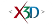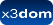## Class: Extrusion

X3D: 3.3
Component: Geometry3DExt
Status:
fully implemented

➨ Fields
➨ Properties

### .nodeTypes. Extrusion

The Extrusion node specifies geometric shapes based on a two dimensional cross-section extruded along a three dimensional spine in the local coordinate system. The cross-section can be scaled and rotated at each spine point to produce a wide variety of shapes.

### HTML Encoding and Default Values⤴

``` <Extrusion beginCap='true' ccw='true' convex='true' creaseAngle='0' crossSection='[(1,1), (1, -1), (-1, -1), (-1, 1), (1, 1)]' endCap='true' height='0' lit='true' metadata='X3DMetadataObject' orientation='[(0,0,0,1)]' scale='[(1,1)]' solid='true' spine='[(0,0,0)]' useGeoCache='true' ></Extrusion> ```

### Constructor⤴

#### Extrusion(ctx)⤴

Constructor for Extrusion

##### Parameters:
Name Type Argument Default Description
`ctx` Object <optional>
null context object, containing initial settings like namespace

### Fields⤴

These are the X3D / X3DOM fields of this node. Values should usually be received / set as strings via DOM functions (i.e., using `setAttribute("myFieldName", "myFieldValue")` and `getAttribute("myFieldName")`).
Name Type Default Value Range Inheritance Standard Description
beginCap SFBool trueSpecifies whether the beginCap should exist.
ccw SFBool true X3DGeometryNodeThe ccw field defines the ordering of the vertex coordinates of the geometry with respect to user-given or automatically generated normal vectors used in the lighting model equations.
convex SFBool trueThe convex field indicates whether all polygons in the shape are convex.
creaseAngle SFFloat 0 [0, inf]The creaseAngle field affects how default normals are generated. If the angle between the geometric normals of two adjacent faces is less than the crease angle, normals shall be calculated so that the faces are shaded smoothly across the edge; otherwise, normals shall be calculated so that a lighting discontinuity across the edge is produced. Crease angles shall be greater than or equal to 0.0 angle base units.
crossSection MFVec2f [(1,1), (1, -1), (-1, -1), (-1, 1), (1, 1)]Describes the cross section as a 2D piecewise linear curve (series of connected vertices).
endCap SFBool trueSpecifies whether the endCap should exist.
height SFFloat 0 [0, inf]Convenience field for setting default spine.
lit SFBool true X3DGeometryNodeSpecifies whether this geometry should be rendered with or without lighting.
metadata SFNode X3DMetadataObject X3DNodeField to add metadata information
orientation MFRotation [(0,0,0,1)]Defines an array of orientations for each extrusion step.
scale MFVec2f [(1,1)] [0, inf]Defines an array of 2D scale values for each extrusion step.
solid SFBool true X3DGeometryNodeSpecifies whether backface-culling is used. If solid is TRUE only front-faces are drawn.
spine MFVec3f [(0,0,0)]Describes the spine as a 3D piecewise linear curve (series of conntected vertices).
useGeoCache SFBool true X3DGeometryNodeMost geo primitives use geo cache and others might later on, but one should be able to disable cache per geometry node. Defaults to global useGeoCache setting parameter.